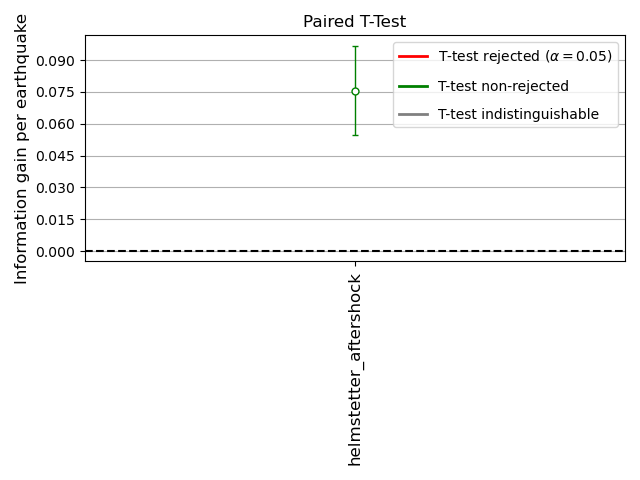# Grid-based Forecast Evaluation

This example demonstrates how to evaluate a grid-based and time-independent forecast. Grid-based forecasts assume the variability of the forecasts is Poissonian. Therefore, Poisson-based evaluations should be used to evaluate grid-based forecasts.

Overview:
1. Define forecast properties (time horizon, spatial region, etc).

2. Obtain evaluation catalog

3. Apply Poissonian evaluations for grid-based forecasts

4. Store evaluation results using JSON format

5. Visualize evaluation results

Most of the core functionality can be imported from the top-level `csep` package. Utilities are available from the `csep.utils` subpackage.

```import csep
from csep.core import poisson_evaluations as poisson
from csep.utils import datasets, time_utils, plots

# Needed to show plots from the terminal
import matplotlib.pyplot as plt
```

## Define forecast properties

We choose a Time-independent Forecast to show how to evaluate a grid-based earthquake forecast using PyCSEP. Note, the start and end date should be chosen based on the creation of the forecast. This is important for time-independent forecasts because they can be rescale to any arbitrary time period.

```from csep.utils.stats import get_Kagan_I1_score

start_date = time_utils.strptime_to_utc_datetime('2006-11-12 00:00:00.0')
end_date = time_utils.strptime_to_utc_datetime('2011-11-12 00:00:00.0')
```

For this example, we provide the example forecast data set along with the main repository. The filepath is relative to the root directory of the package. You can specify any file location for your forecasts.

```forecast = csep.load_gridded_forecast(datasets.helmstetter_aftershock_fname,
start_date=start_date,
end_date=end_date,
name='helmstetter_aftershock')
```

We will download the evaluation catalog from ComCat (this step requires an internet connection). We can use the ComCat API to filter the catalog in both time and magnitude. See the catalog filtering example, for more information on how to filter the catalog in space and time manually.

```print("Querying comcat catalog")
catalog = csep.query_comcat(forecast.start_time, forecast.end_time, min_magnitude=forecast.min_magnitude)
print(catalog)
```
```Querying comcat catalog

Start Date: 2007-02-26 12:19:54.530000+00:00
End Date: 2011-02-18 17:47:35.770000+00:00
Min Latitude: 31.9788333 and Max Latitude: 41.1444
Min Longitude: -125.0161667 and Max Longitude: -114.8398
Min Magnitude: 4.96
Found 34 events in the ComCat catalog.

Name: None

Start Date: 2007-02-26 12:19:54.530000+00:00
End Date: 2011-02-18 17:47:35.770000+00:00

Latitude: (31.9788333, 41.1444)
Longitude: (-125.0161667, -114.8398)

Min Mw: 4.96
Max Mw: 7.2

Event Count: 34
```

We need to remove events in the evaluation catalog outside the valid region specified by the forecast.

```catalog = catalog.filter_spatial(forecast.region)
print(catalog)
```
```Name: None

Start Date: 2007-02-26 12:19:54.530000+00:00
End Date: 2011-02-18 17:47:35.770000+00:00

Latitude: (31.9788333, 41.1155)
Longitude: (-125.0161667, -115.0481667)

Min Mw: 4.96
Max Mw: 7.2

Event Count: 32
```

## Compute Poisson spatial test

Simply call the `csep.core.poisson_evaluations.spatial_test()` function to evaluate the forecast using the specified evaluation catalog. The spatial test requires simulating from the Poisson forecast to provide uncertainty. The verbose option prints the status of the simulations to the standard output.

```spatial_test_result = poisson.spatial_test(forecast, catalog)
```

## Store evaluation results

PyCSEP provides easy ways of storing objects to a JSON format using `csep.write_json()`. The evaluations can be read back into the program for plotting using `csep.load_evaluation_result()`.

```csep.write_json(spatial_test_result, 'example_spatial_test.json')
```

## Plot spatial test results

We provide the function `csep.utils.plotting.plot_poisson_consistency_test()` to visualize the evaluation results from consistency tests.

```ax = plots.plot_poisson_consistency_test(spatial_test_result,
plot_args={'xlabel': 'Spatial likelihood'})
plt.show()
```## Plot ROC Curves

We can also plot the Receiver operating characteristic (ROC) Curves based on forecast and testing-catalog. In the figure below, False Positive Rate is the normalized cumulative forecast rate, after sorting cells in decreasing order of rate. The “True Positive Rate” is the normalized cumulative area. The dashed line is the ROC curve for a uniform forecast, meaning the likelihood for an earthquake to occur at any position is the same. The further the ROC curve of a forecast is to the uniform forecast, the specific the forecast is. When comparing the forecast ROC curve against an catalog, one can evaluate if the forecast is more or less specific (or smooth) at different level or seismic rate.

Note: This figure just shows an example of plotting an ROC curve with a catalog forecast.

```print("Plotting ROC curve")
_ = plots.plot_ROC(forecast, catalog)
``````Plotting ROC curve
```

## Calculate Kagan’s I_1 score

We can also get the Kagan’s I_1 score for a gridded forecast (see Kagan, YanY.  Testing long-term earthquake forecasts: likelihood methods and error diagrams, Geophys. J. Int., v.177, pages 532-542).

```I_1 = get_Kagan_I1_score(forecast, catalog)
print("I_1score is: ", I_1)
```
```I_1score is:  [2.31435371]
```

Total running time of the script: (0 minutes 7.701 seconds)

Gallery generated by Sphinx-Gallery